## How to Convert Angles from Radians to Degrees in Excel

This post will guide you how to convert angles from radians to degrees with a formula in Excel. How do I convert angle between radians to degrees in Excel.

When you want to solve trigonometric expressions like sine, cosine and tangent, you need to use radians to perform these calculations. So if your have a range of list that contain degree values, and you have to convert it to radians for calculation.

You may be have a list of data that contain radian values, and you want to convert it from radians to degrees. You can use a build-in function known as RADIANS function, it can be used to convert degrees to radians. And you can use the DEGREES function to convert radians into degrees in Excel.

## Convert angles from Radians to degrees

Assuming that you have a list of degrees in range B1:B4, and you want to convert those values into radians, just use the following formula:

`=RADIANS(B1)`

Type this formula into a blank cell or the adjacent cell C1, press Enter key to apply this formula. And then drag the AutoFill Handle down to other cells until to C4.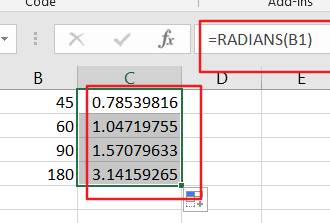You would see that all degree values has been converted to radian values.

## Convert Angles from Degrees to Radians

If you want to convert radian values to degree values, just use the below formula based on the DEGREES function.

`=DEGREEES(C1)`

Type this formula into a blank cell or the adjacent cell D1, press Enter key to apply this formula. And then drag the AutoFill Handle down to other cells until to D4.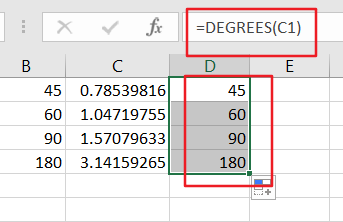You would see that all radian values have been converted to degree values.

### Related Functions

• Excel DEGREES Function
The Excel DEGREES function converts radians into degrees. And it will return a numeric value.The syntax of the DEGREES function is as below:=DEGREES (angle)…

## Excel COS Function

This post will guide you how to use Excel COS function with syntax and examples in Microsoft excel.

### Description

The Excel COS function returns the cosine of a given angle. If you want to supply an angle to COS function in degrees, then you need to multiply the angle by PI()/180. Or you can use the RADIANS function to the angle to radians.

The COS function is a build-in function in Microsoft Excel and it is categorized as a Math and Trigonometry Function.

The COS function is available in Excel 2016, Excel 2013, Excel 2010, Excel 2007, Excel 2003, Excel XP, Excel 2000, Excel 2011 for Mac.

### Syntax

The syntax of the COS function is as below:

`= COS(number)`

Where the COS function argument is:

• number –This is a required argument. A number that used to be calculate the cosine of an angle.

Note:

• If your argument is in degrees, multiply it by PI()/180 or use the RADIANS function to convert it to radians.

### Excel COS Function Examples

The below examples will show you how to use Excel COS Function to return the cosine of an angle.

1# get the cosine of 1.25 radians, enter the following formula in Cell B1.

`=COS(1.25)`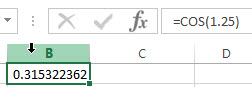2# get the cosine of 45 degrees, enter the following formula in Cell B2.

`=COS(45*PI()/180)`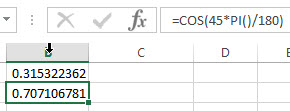3# get the cosine of 30 degrees, enter the following formula in Cell B3.

`=COS(RADIANS(30))`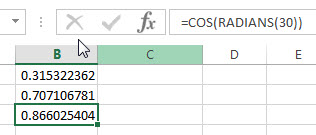### Related Functions

• Excel SIN function
The Excel TANH function returns the hyperbolic tangent of a given number.The syntax of the TANH function is as below:=TANH (number)…
• Excel ACOS function
The Excel ACOS function returns the arccosine value of a number.The ACOS function is a build-in function in Microsoft Excel and it is categorized as a Math and Trigonometry Function.The syntax of the ACOS function is as below:= ACOS(number)…
• Excel ACOSH function
The Excel ACOSH function returns the inverse hyperbolic cosine of a number.The syntax of the ACOSH function is as below:= ACOSH (number)…
• Excel ASIN function
The Excel ASIN function returns the arcsine value of a number.The syntax of the ASIN function is as below:= ASIN (number)…
• Excel PI function
The Excel PI function returns the value of mathematical constant PI. The returned value is 3.14159265358979 and ti will accurate to 15 digits.The syntax of the PI function is as below:=PI()…
• Excel TAN function
The Excel TAN function returns the tangent of a given angle. So you can use this function to calculate the tangent of an angle in radians. The syntax of the TAN function is as below:=TAN (number)…

## Excel SIN Function

This post will guide you how to use Excel SIN function with syntax and examples in Microsoft excel.

### Description

The Excel SIN function returns the sine of a given angle. If you want to supply an angle to SIN function in degrees, then you need to multiply the angle by PI()/180. Or you can use the RADIANS function to the angle to radians.

The SIN function is a build-in function in Microsoft Excel and it is categorized as a Math and Trigonometry Function.

The SIN function is available in Excel 2016, Excel 2013, Excel 2010, Excel 2007, Excel 2003, Excel XP, Excel 2000, Excel 2011 for Mac.

### Syntax

The syntax of the SIN function is as below:

`=SIN (number)`

Where the SIN function argument is:

• number –This is a required argument. A number that used to be calculate the sine of an angle.

Note:

• If your argument is in degrees, multiply it by PI()/180 or use the RADIANS function to convert it to radians.

### Excel SIN Function Examples

The below examples will show you how to use Excel SIN Function to return the sine of an angle.

1# get the sine of pi radians, enter the following formula in Cell B1.

`=SIN(PI())`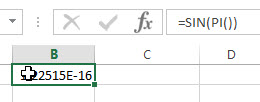2# get the sine of pi/2 radians, enter the following formula in Cell B2.

`=SIN(PI()/2)`3# get the sine of 45 degrees, enter the following formula in Cell B3.

`=SIN(45*PI()/180)`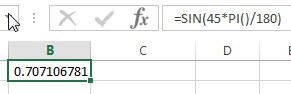Or using the another formula to get the same result as follow:

`=SIN(RADIANS(45))`### Related Functions

• Excel COS function
The Excel COS function returns the cosine of a given angle. The syntax of the COS function is as below:= COS(number)…
• Excel ACOS function
The Excel ACOS function returns the arccosine value of a number.The ACOS function is a build-in function in Microsoft Excel and it is categorized as a Math and Trigonometry Function.The syntax of the ACOS function is as below:= ACOS(number)…
• Excel ACOSH function
The Excel ACOSH function returns the inverse hyperbolic cosine of a number.The syntax of the ACOSH function is as below:= ACOSH (number)…
• Excel ASIN function
The Excel ASIN function returns the arcsine value of a number.The syntax of the ASIN function is as below:= ASIN (number)…
• Excel PI function
The Excel PI function returns the value of mathematical constant PI. The returned value is 3.14159265358979 and ti will accurate to 15 digits.The syntax of the PI function is as below:=PI()…
• Excel TAN function
The Excel TAN function returns the tangent of a given angle. So you can use this function to calculate the tangent of an angle in radians. The syntax of the TAN function is as below:=TAN (number)…

## Excel TAN Function

This post will guide you how to use Excel TAN function with syntax and examples in Microsoft excel.

Description

The Excel TAN function returns the tangent of a given angle. So you can use this function to calculate the tangent of an angle in radians. If you want to put an angle to TAN in degrees, you need to multiply the angle by PI()/180, then put the returned result into the TAN function. Or you can use the RADIANS function to combine with the TAN function.

The TAN function is a build-in function in Microsoft Excel and it is categorized as a Math and Trigonometry Function.

The TAN function is available in Excel 2016, Excel 2013, Excel 2010, Excel 2007, Excel 2003, Excel XP, Excel 2000, Excel 2011 for Mac.

Syntax

The syntax of the TAN function is as below:

`=TAN (number)`

Where the TAN function argument is:

• number -This is a required argument. A numeric value that used to calculate the tangent of an angle in radians.

Note:

• If your argument is in degrees, multiply it by PI()/180 or use the RADIANS function to convert it to radians.

Excel TAN Function Examples

The below examples will show you how to use Excel TAN Function to return the tangent of an angle in radians.

1# get the tangent of 0.55 radians, enter the following formula in Cell B1.

`=TAN(0.55)`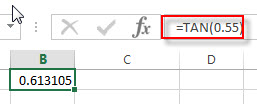2# get the tangent of 39 degrees, use the below formula in Cell B2

`=TAN(39*PI()/180)`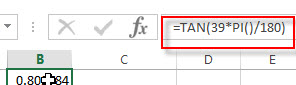Or you can use the RADINANS function to convert the angle to radians, then pass the returned value into TAN function to achieve the same result. Type the following formula in Cell B3:

`=TAN(RADIANS(39))`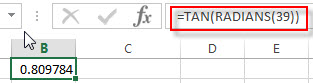### Related Functions

• Excel TANH function
The Excel TANH function returns the hyperbolic tangent of a given number.The syntax of the TANH function is as below:=TANH (number)…
• Excel SIN function
The Excel TANH function returns the hyperbolic tangent of a given number.The syntax of the TANH function is as below:=TANH (number)…
• Excel ACOS function
The Excel ACOS function returns the arccosine value of a number.The ACOS function is a build-in function in Microsoft Excel and it is categorized as a Math and Trigonometry Function.The syntax of the ACOS function is as below:= ACOS(number)…
• Excel ACOSH function
The Excel ACOSH function returns the inverse hyperbolic cosine of a number.The syntax of the ACOSH function is as below:= ACOSH (number)…
• Excel ASIN function
The Excel ASIN function returns the arcsine value of a number.The syntax of the ASIN function is as below:= ASIN (number)…
• Excel ATAN function
The Excel ATAN function returns the arctangent of a number. So this function can be used to calculates the arctangent of a supplied number. The returned angle is given in radians in the range –pi/2 to pi/2. The syntax of the ATAN function is as below:= ATAN (number)…
• Excel ATAN2 function
The Excel ATAN2 function returns the arctangent, or inverse tangent, of the specified x- and y-coordinates. And you can use the ATAN2 function to calculate the arctangent of a given x and y coordinates. The syntax of the ATAN2 function is as below:= ATAN2 (x_num, y_num)…
• Excel ATANH function
The Excel ATANH function returns the inverse hyperbolic tangent of a given number. The syntax of the ATANH function is as below:= ATANH (number)…
• Excel PI function
The Excel PI function returns the value of mathematical constant PI. The returned value is 3.14159265358979 and ti will accurate to 15 digits.The syntax of the PI function is as below:=PI()…